# Simple proportion + ratio - math problems

#### Number of problems found: 52

• CandiesThere are red, blue and green candies in bad. Red to green is in 6:11 ratio and blue to red in a 7: 5 ratio. In what proportion are blue to green candies?
• Proportion 3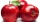For every 8 mango trees in the orchard, there are 4-star apple trees. If there are 1320 trees, how many trees of each kind are there?
• RatiosReduce the numbers: 50 in a 1:2 ratio 111 at a ratio of 2:3 70 at 10:50 560 at a ratio of 3:8
• A mapA map of size 60cm by 25cm is reduced in the ratio of 3:5, twice. What are the new dimensions?
• Gold inheritance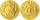The king divided the inheritance with his three sons in the ratio of 7: 6: 4. Two of them received 286,000 gold. How much each of the sons got.
• Proportional relationship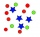The ordered pairs (6,24) and (1, s) represent a proportional relationship. Find the value of s.
• Mixture of nuts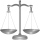Alice bought a mixture of nuts containing cashew nuts, hazelnuts and peanuts in a ratio of 1: 2: 3. Calculate the weight in grams of the whole mixture if the peanuts weigh 90 g.
• Five mechanicsFive mechanics will make a car for some time. How many cars can 30 mechanics produce at the same time?
• Rails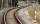18 m railway weighs 1260 kg. How much weighs 100 m of welded railways?
• Two brothersThe two brothers were to be divided according to the will of land at an area of 1ha 86a 30m2 in a ratio of 5:4. How many will everyone get?
• PaintersTen painters paint the school in 20 days. How many days do four painters paint the school at the same pace of work?
• PumpsFive pumps pumped 1,800 hl of water for 3 hours. How many hectoliters of water pump same two powerful pumps for six hours?
• R1+R2 in parallelThe resistance ratio of the first and second resistors is 3: 1 and the current flowing through the first resistor is 300 mA. What current flows through the second resistor (resistors connected in parallel)?
• The straight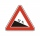The straight path rises by 72 cm every 3 m of its length. How many meters will it climb to 350 m?
• Six percents6% of the base is 21. How much is 28% of this base?
• Two villages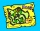On the map with a scale of 1:40000 are drawn two villages actually 16 km away. What is their distance on the map?
• Everyone drinks the same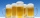24 bricklayers drink 72 beverage bottles a day at the construction site. How many bottles would 19 bricklayers need? Everyone drinks the same.
• Five pumpsThe water tank is filled with two pumps in 48 minutes. How long would it take to fill it with 5 same pumps?
• Snack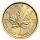CZK 450 was paid for a snack for 30 pupils. How many CZK will we pay for the same snack for 28 pupils?
• Save trees25 tons of old paper will save 1,600 trees. How many tons of paper is needed to save the 32 trees in the park?

Do you have an interesting mathematical word problem that you can't solve it? Submit a math problem, and we can try to solve it.

We will send a solution to your e-mail address. Solved examples are also published here. Please enter the e-mail correctly and check whether you don't have a full mailbox.

Please do not submit problems from current active competitions such as Mathematical Olympiad, correspondence seminars etc...

Check out our ratio calculator. Simple proportion - math problems. Ratio - math problems.